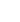15min:
EXACT QUANTUM DYNAMICS CALCULATIONS USING PHASE SPACE WAVELETS.

THOMAS HALVERSON, BILL POIRIER, Department of Chemistry and Biochemistry, and Department of Physics, Texas Tech University, P.O. Box 41061, Lubbock TX, USA.

In a series of earlier papers, the authors introduced the first exact quantum dynamics method that defeats the exponential scaling of CPU effort with system dimensionality. The method used a ``weylet'' basis set (orthogonalized Weyl-Heisenberg wavelets), combined with a phase space truncation scheme first proposed by M. Davis and E. Heller. Here, we use a related, but much simpler, wavelet basis consisting of momentum-symmetrized phase space Gaussians. Despite being non-orthogonal, symmetrized Gaussians exhibit collective locality, allowing for effective phase space truncation and the defeat of exponential scaling. A ``universal'' and remarkably simple code has been written, which is dimensionally independent, and which also exploits massively parallel algorithms. The codes have been used to calculate the vibrational spectra of several molecules of varying dimensionality.